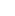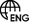Inquiry CartInquiry CartSwitch Language
Back
Select Language

# Thinking about the capacity limits of WDM systems

Introduction: What is the capacity limit of WDM?

This is not merely a theoretical calculation, but a conclusion that can only be reached after taking into account the effects of three major factors, including the available spectrum of the fiber, the maximum transmission rate at a single wavelength, and the minimum allowable wavelength gap.

Ascent Optics can provide products for WDM MUX DEMUX applications.

Before stating the WDM capacity again, it is necessary to understand the concepts of bandwidth and transmission rate. The concept of bandwidth comes from the definition of the signal frequency band, while the transmission rate corresponds to the information rate in the effectiveness of the quality indicators of data communication systems.

## Bandwidth Quotes

Bandwidth refers to the amount of data that can be transmitted at a fixed time, i.e., the capacity of the data that can be transmitted in the transmission pipeline.

In digital devices, the bandwidth is usually expressed in bits per second(bps), which represents the number of bits per second that can be transmitted; in analog devices, the bandwidth is typically expressed in cycles per second or Hertz (Hz).

“Bandwidth” (bandwidth) has the following two different meanings.

1. refers to the width of the signal frequency band. The bandwidth of a signal is the frequency range occupied by the various different frequency components contained in the signal.

2. In computer networks, bandwidth is used to indicate the capacity of the network’s communication lines to transmit data, so the network bandwidth indicates the “maximum data rate” that can be passed from one point in the network to another in a unit of time.

## 1. Bandwidth concept

For a signal, the frequency range between zero frequency and the highest frequency component to be considered is called the bandwidth.

1.1 Frequency band of the signal

(i) In practice, for a spectrum with the sampling function as the envelope, the frequency range between the zero frequency and the frequency corresponding to the first crossing of the zero point of the spectral envelope is defined as the signal frequency band.

(ii) For the general spectrum, the frequency range starting from zero frequency and the frequency where the amplitude drops to 1/10 of the maximum point of the envelope is defined as the signal frequency band.

## 2. Transmission rate

2.1 Quality indicators of data communication system

In summary, there are several aspects.

Validity: refers to the speed of transmission of information.

Reliability: refers to the quality of transmission of information.

Adaptability: refers to environmental use conditions.

Standardization: refers to the standard interchangeability of components.

Economy: refers to the level of cost.

Maintenance: ease of use.

The concepts described below are all important indicators of effectiveness.

2.2 Code element rate

Definition: The number of transmitted signal elements per second is called the code element rate. Unit: Baud (B), denoted by the symbol R.B .

The code element rate is solely determined by the code element width T. For instance, in an N-decimal signal, the code element width is T, and the number of code elements per second is 1/T, so the code element rate RB = 1/T baud, code element rate is also called modulation rate. The modulation rate represents the shortest time duration within the signal element.

2.3 Information rate

Definition: The amount of information transmitted per second is called the information rate of the data signal. Unit: bits per second (bit/s), expressed by the symbol R.b .

An N-decimal data signal, there are N possible states for each code element, and let the probability P of each state occurring be the same, i.e., P=1/N. In information theory, the amount of information in each code element is defined as

I=log2 1/P=log2 N(bit)

An N-decimal data signal with an information rate of

Rb =RB ×I=RB log2 N(bit/s)

For binary data signals, the code element rate and the data information rate are numerically equal, but their units are different.

Taking into account the above description, consider, for example, the available bandwidth of a signal with 155 Mbit/s rate , which should be numerically equal to 155MHZ. For binary data signals, we can establish a correspondence between the transmission number rate and bandwidth.

## 3. Capacity limits of WDM systems

Three main factors are discussed, including the available spectrum of the fiber, the maximum transmission rate at a single wavelength, and the minimum allowable wavelength spacing, in order to derive the possible capacity limit of a single pair of fibers under the current technological constraints. Finally, the theoretical limit of possible capacity of a single pair of fibers is presented once again.

3.1 Available spectra of optical fibers

From the available wavelength range, WDM systems are limited at the low end by the fiber cutoff wavelengths regulated to guard against mode noise, and at the high end by the silica absorption loss and bending loss of the fiber, cable attenuation and fiber dispersion also have some limitations on the operating wavelength range.

According to the latest ITU-T specifications, the available wavelength range are roughly 1260-1675 nm. Deducting the water peak of the fiber at 1385 nm, the total available spectrum is about 415 nm, which corresponds to approximately 58 THZ (usually roughly 50 THZ )【2】.

Of course, the design system will also take into account the light source optical device and other factors, the actual available spectral range will be slightly reduced.

3.2 Maximum transmission rate at a single wavelength

Theoretically, there is an upper limit to the transmission rate of a single wavelength, primarily constrained by factors such as the electron mobility of the silicon and gallium-arsenic materials of the integrated circuit, as well as the by the dispersion and polarization mode dispersion of the transmission medium. Additionally, it is necessary consider whether the developed system’ performance-to-price ratio is cost-effective and has commercial economic value.

From the present point of view, the material issues are not the main limiting factors, especially the indium phosphide material exhibiting excellent performance in the rate above 40Gbit/s, thanks to its high electron and hole mobility. However the dispersion and polarization mode dispersion of the transmission medium, along with  the performance ratio limitations of the system, make the practical prospect of the rate above 40Gbit/s very dim. Therefore, we have reason to consider 40Gbit/s as the maximum transmission rate for a single wavelength.

3.3 Allowable minimum wavelength gap

In theory, the true information bandwidth of an optical signal is approximately twice the transmitted bit rate.

In fact, the minimum wavelength spacing in system design is usually much larger than twice the transmitted bit rate (since optical filters do not have ideal flatness and absolute stability, and light sources also lack absolute stability. Even with wavelength locking techniques, there will still be wavelength drift ).

From a conservative perspective, to estimate the maximum transmission capacity, it is assumed that the minimum wavelength spacing of 2.5 Gbit/s, 10 Gbit/s and 40 Gbit/s is at least 5 times, 2.5 times and 1.25 times the transmission bit rate, corresponding to minimum wavelength spacings of 12.5 GHZ , 25 GHZ and 50 GHZ respectively.

3.4 Capacity limit projection

It is known that the maximum number of wavelengths is 4000, 2000 and 1000 for a single wavelength rate of 2.5Gbit/s, 10Gbit/s and 40Gbit/s at an available spectrum of 50THZ, respectively.

Maximum wavelength number = available spectrum / minimum wavelength gap

From this, the total transmission capacity can be introduced to be around 10Tbit/s, 20Tbit/s and 40Tbit/s respectively

Total transmission capacity = maximum number of wavelengths X single wave rate